You are currently offline. Some features of the site may not work correctly.

# Linear arboricity

In graph theory, a branch of mathematics, the linear arboricity of an undirected graph is the smallest number of linear forests its edges can be… Expand
Wikipedia

## Papers overview

Semantic Scholar uses AI to extract papers important to this topic.
2013
2013
• Discrete Math., Alg. and Appl.
• 2013
• Corpus ID: 207153446
A linear k-forest of an undirected graph G is a subgraph of G whose components are paths with lengths at most k. The linear k… Expand
Is this relevant?
2012
2012
• Journal of Graph Theory
• 2012
• Corpus ID: 1158069
The linear arboricity la(G) of a graph G is the minimum number of linear forests (graphs where every connected component is a… Expand
•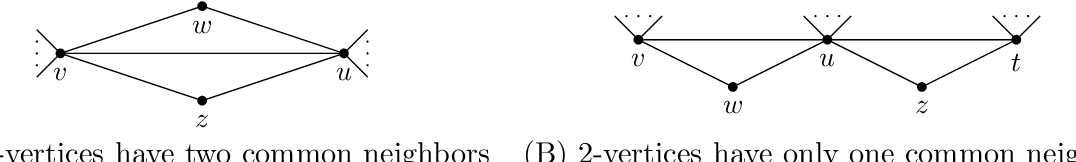•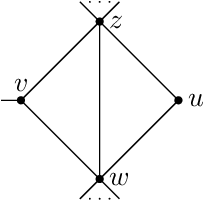•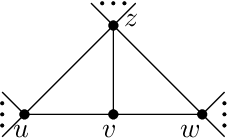•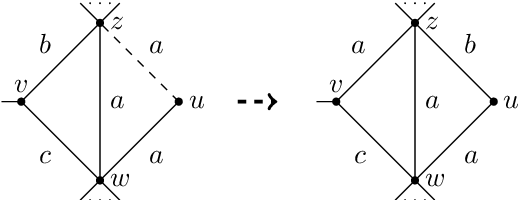•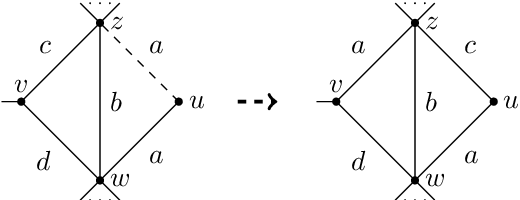Is this relevant?
2007
2007
• Theor. Comput. Sci.
• 2007
• Corpus ID: 5831011
The linear arboricity of a graph G is the minimum number of linear forests which partition the edges of G. Akiyama, Exoo and… Expand
Is this relevant?
2006
2006
• Discret. Math.
• 2006
• Corpus ID: 36178651
A distance graph is a graph G(R,D) with the set of all points of the real line as vertex set and two vertices u,[email protected… Expand
Is this relevant?
2001
2001
• Graphs and Combinatorics
• 2001
• Corpus ID: 5175497
Abstract. We find upper bounds on the linear k-arboricity of d-regular graphs using a probabilistic argument. For small k these… Expand
•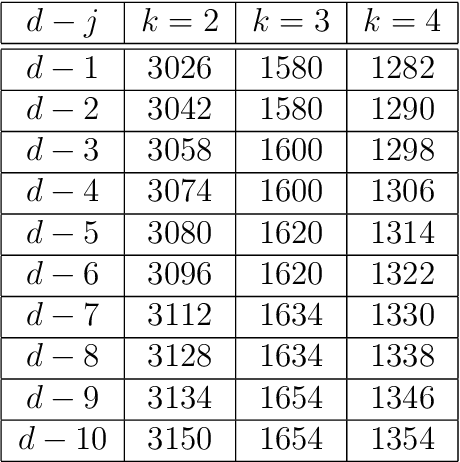Is this relevant?
1988
1988
The point-linear arboricity of a graph G=(V, E), written as p 0 (G), is defined as p 0 (G)=min{k | there exists a partition of V… Expand
•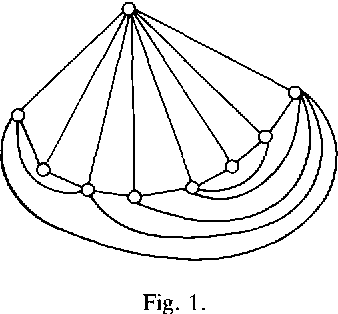Is this relevant?
Highly Cited
1988
Highly Cited
1988
AbstractAlinear forest is a forest in which each connected component is a path. Thelinear arboricity la(G) of a graphG is the… Expand
Is this relevant?
1987
1987
• Networks
• 1987
• Corpus ID: 27519621
A linear diforest is a digraph whose connected components are paths. We define the linear arboricity of a digraph, denoted la(G… Expand
Is this relevant?
1982
1982
• Discret. Math.
• 1982
• Corpus ID: 30129358
We present here a conjecture on partitioning the edges of a graph into k-linear forests (forest whose connected components are… Expand
Is this relevant?
1981
1981
• Networks
• 1981
• Corpus ID: 13628070
The linear arboricity of a graph is the minimum number of linear forests into which its lines can be decomposed. We find that the… Expand
Is this relevant?